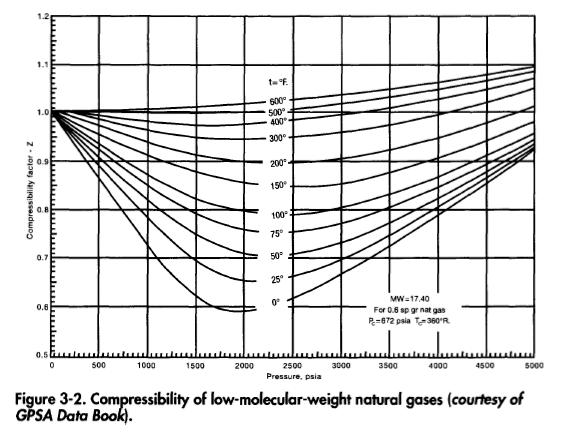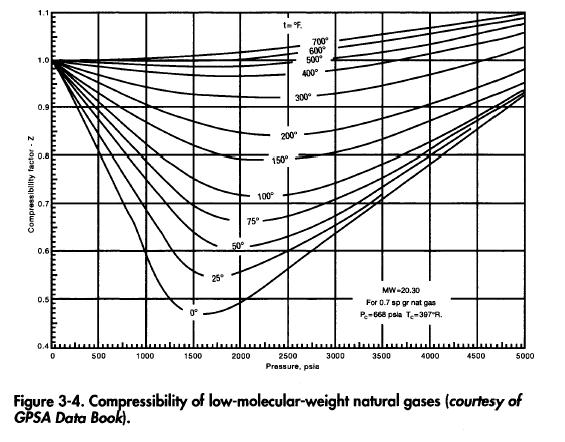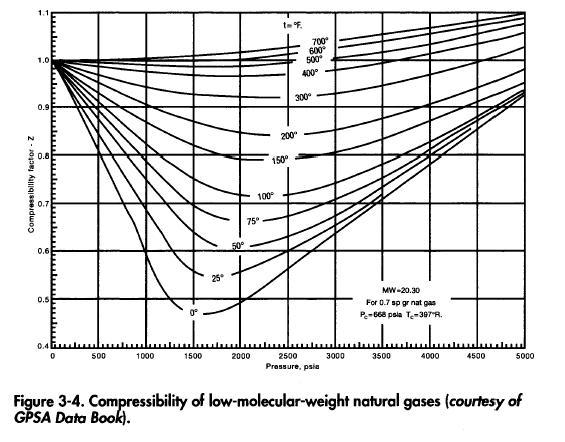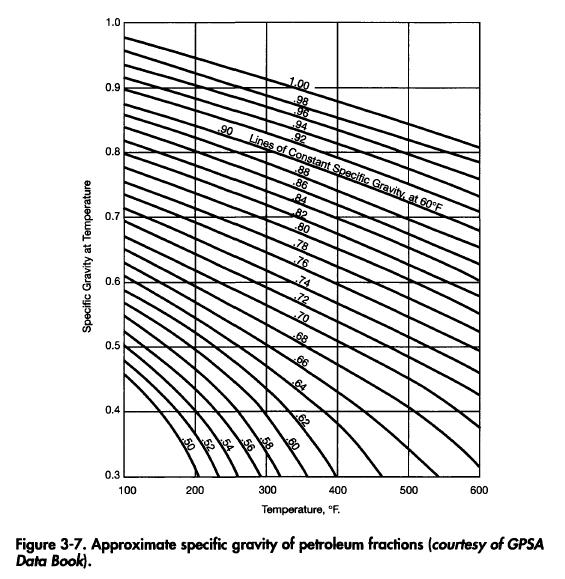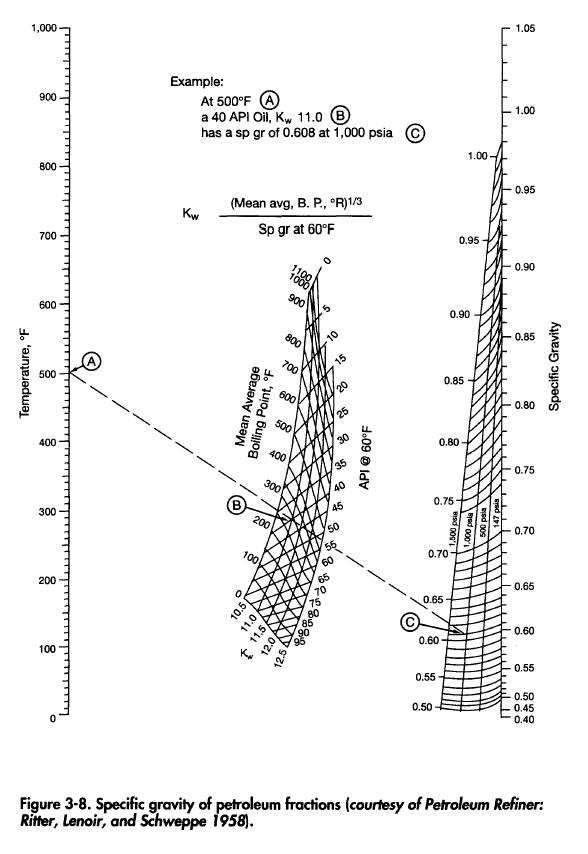﻿ Specific Gravity and Density | Oil & Gas Process Engineering

## Specific Gravity and Density

Specific gravity of a liquid is the ratio of the density of the liquid at 60°F to the density of pure water. API gravity is related to the specific gravity by the following equation:The specific gravity of a gas is the ratio of the density of the gas to the density of air at standard conditions of temperature and pressure. It may be related to the molecular weight by the following equation: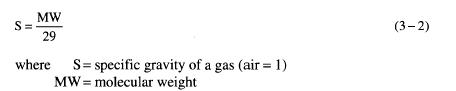In most calculations the specific gravity of the gas is always referred to in terms of standard conditions of temperature and pressure and therefore is always given by Equation 3-2 once the molecular weight of the gas is known. The density of a gas at any condition of temperature and pressure can be determined by remembering that the density of air at standard conditions of temperature and pressure (60°F and 14.7 psia) is 0.0764 lb/ft3. The density of gas is thus given as: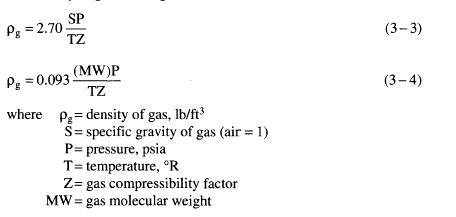Derivation of Equations 3-3 and 3-4 Density of gas at standard conditions of temperature and pressure is by definition: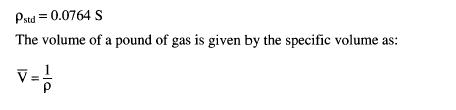The equation of state for a gas is given for engineering calculations as: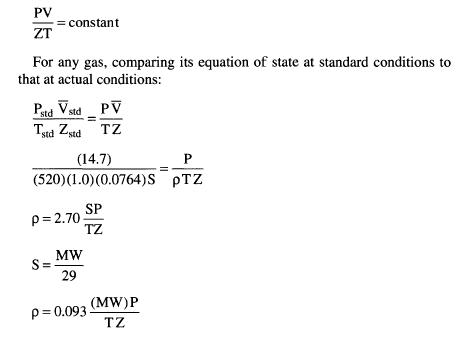The compressibility factor for a natural gas can be approximated from Figures 3-1 through 3-6, which are from the Engineering Data Book of the Gas Processor Suppliers Association.

In most calculations the specific gravity of liquids is normally referenced to actual temperature and pressure conditions. Figures 3-7 and 3-8 can be used to approximate how the specific gravity of a liquid decreases with increasing temperature, assuming no phase changes. In most practical pressure drop calculations associated with production facilities, the difference in specific gravity caused by pressure changes will not be severe enough to be considered if there are no phase changes.

For hydrocarbons, which undergo significant phase changes, Figure 3-8 can be used as an approximation of the specific gravity at a given pressure and temperature, once the API gravity of the liquid is known.

It should be pointed out that both Figures 3-7 and 3-8 are approximations only for the liquid component. Where precise calculation is required for a hydrocarbon, it is necessary to consider the gas that is liberated with decreasing pressure and increasing temperature. Thus, if a hydrocarbon is heated at constant pressure, its specific gravity will increase as the lighter hydrocarbons are liberated. The change in the molecular makeup of the fluid is calculated by a “flash calculation,” which is described in more detail later.